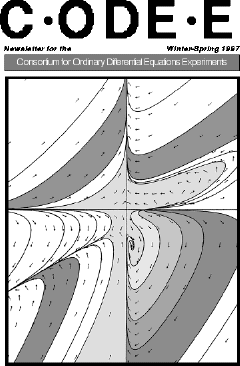Course Title: Ordinary Differential Equations MATH 6410 - 1 Andrejs Treibergs http://www.math.utah.edu/~treiberg/M6410.html M, W, F, 2:00 - 2:50 in LCB 215 10:40-11:30 M, W, F, in JWB 224 (tent.) treiberg@math.utah.edu Math 5210 or consent of instructor. Carmen Chicone, Ordinary Differential Equations with Applications Springer Texts in Applied Mathematics 34, 1999.In this first semester of a year long graduate course in differential equations, we shall focus on ordinary differential equations and dynamical systems. The second semester, Math 6420, will emphasize partial differential equations. In this course, along with the Math 6420, we shall try to cover the syllabus for the qualifying exam in differential equations. Although some mathematical sophistication is required to take the course, we shall provide any backgroung materials needed by the class.

We shall follow Chicone's text covering existence and uniqueness of solutions of ordinary differential equations, continuous dependence on data, stability theory, Floquet theory, invariant manifolds. We shall discuss as many applications as we can. Topics include (depending on time):

• Introduction to ODE. Applications. Review of calculus.
• Linear systems and stability.
• Existence, uniqueness and continuity theorems.
• Qualitative theory, Lipunov stability. Limit sets and attractors.
• Applications to physical and biological systems. Charged particle, coupled pendula, planetary systems.
• Invariant manifolds and linearizations. Hartman-Grobman theorem.
• Planar flows. Poincaré-Bendixon theory.
• Periodic solutions and their stability.
• Sturm-Liouville Theory.

Last updated: 5 / 6 / 8